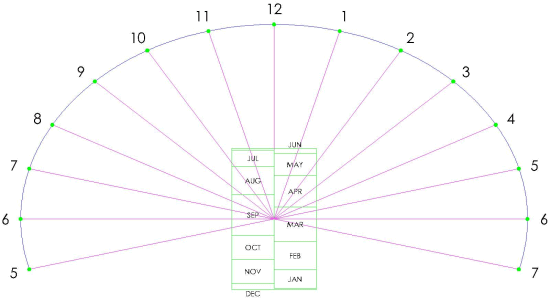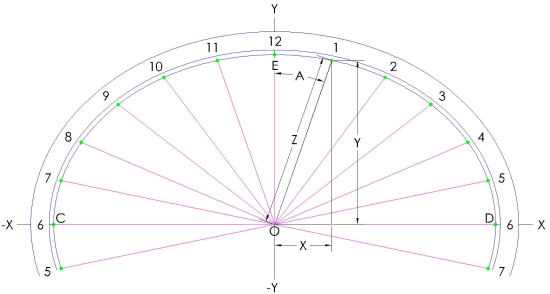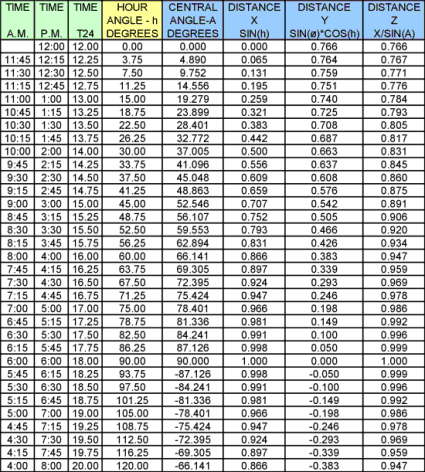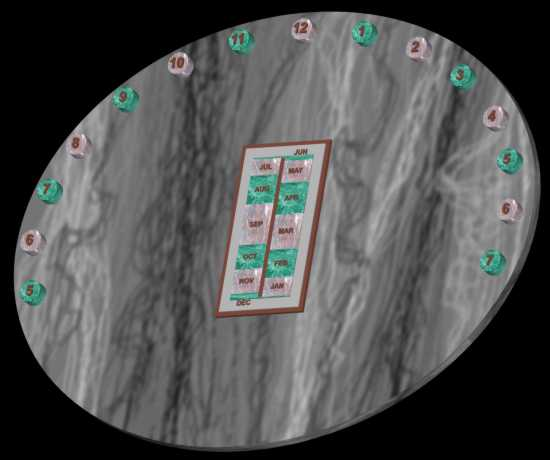The Sundial Primer created by Carl SabanskiThe Sundial Primer Index
 Analemmatic Sundial Analemmatic Sundial: sundials consisting of hour points (rather than hour lines) laid round an ellipse, and a movable gnomon perpendicular to the dial plane. It may be on any plane, but the most usual form is horizontal. In the horizontal version of the dial, gnomon position lies on the straight North-South minor axis, at a point determined by the sun's declination (i.e. the date). The analemmatic dial may be regarded as a projection of the universal equatorial ring dial. Azimuthal or Azimuth Sundial: any dial which uses the sun's azimuth for indicating the time. It usually needs to be aligned N-S, and has a vertical style (if it has no dependence on altitude).Declination (of the Sun): the angular distance of the Sun above or below the celestial equator. Its value follows an annual sine wave like curve, varying between 0° at the equinoxes and ±23.4° (approx.) at the solstices. It has positive values when the Sun is above the celestial equator (summer in the Northern hemisphere) and negative when below. Hour Point: a point on a dial plane indicating the crossing of the gnomon's shadow at a particular time. Hour points replace hour lines on dials where the shadow edge does not pass through the dial centre. The analemmatic sundial presented here is a horizontal azimuth sundial with a moveable vertical gnomon. It uses the azimuth of the sun to indicate the time. The determination of the hour points on the ellipse requires that you know the latitude (ø) where the sundial is to be located. To calculate the dimensions of the scale of dates you will need to know the sun's declination on particular dates for each month. Figure 1 illustrates an analemmatic sundial with its scale of dates.Figure 1: Analemmatic Sundial (CAD) Figure 2 illustrates the design layout for the sundial. It is assumed that the semi-major axis of the ellipse, OC or OD and designated as M, is 1. When the calculations are complete a series of numbers will be established that will be multiplied by the actual semi-major axis dimension to establish the position of the hour points. The semi-minor axis of the ellipse, m, is the line OE and determined as follows.m = sin ø where ø is the latitude.There will be two methods for positioning the hour points available. These include: The horizontal dimension "X" that lies on the east-west line and the vertical dimension "Y" that lies on the north-south line. The central angle "A" and the dimension "Z".Figure 2: Analemmatic Sundial Layout (CAD) The horizontal dimension "X" from point "O" is:X = sin h where h is the hour angle, in degrees, given by:h = (T24 - 12) * 15°and T24 is the time in 24-hour clock notation (hours after midnight) in decimal hours.The vertical dimension "Y" from point "O" is:Y = sin ø * cos hNote that the calculation of "X" only requires the hour angle and would be the same for a dial at any latitude. The dimension "Y" is dependent upon the latitude.The central angle "A" is:A = arctan {(tan h) / (sin ø)}The dimension "Z" from point "O" to the hour point, in this example 1, is:Z = (sin h) / (sin A)Table 1 shows the calculation performed for a sundial located at latitude 50°N. Notice that the hour line angles for the am and pm hours are symmetrical about the noon hour line. Click here to download a spreadsheet that will perform these calculations for you.Table 1: Analemmatic Sundial Hour PointsThe next step is to establish the scale of dates. This is the scale that is used to determine the position of the gnomon on a given day of the year. The scale point is determined for the first day of each month and for the two extremes at the time of the solstices. To do this, the sun's declination is required for each of these dates. The values used were obtained from "The Dialist's Companion". The scale of dates is determined as follows:S = tan (dec) * cos øwhere "dec" is the sun's declination.In Table 2 the calculation is performed for the same sundial. The spreadsheet offered above will perform this calculation if you wish to use different days or different declination values.Table 2: Analemmatic Sundial Scale of DatesThe layout of the scale of dates begins at point "O". Positive numbers are measured up the "Y" axis and negative numbers down the "-Y" axis. Figure 3 illustrates a layout for the scale of dates.Figure 3: Scale of DatesNow that the multipliers are established all that is required is to establish the length of the semi-major axis. Remember that if you are building an interactive sundial, where a person acts as the gnomon, you do not want the dial to be of unreasonable size or it will be difficult to determine where the shadow is pointing. Once done, then you can calculate all the dimensions required to lay out the hour points and the scale of dates.For an image complete with shadow click here.